The peak voltage in the output of a half-wave diode rectifier fed with a sinusoidal signal without filter is 10 V. The dc component of the output voltage is
(a)$10/\sqrt{2}$  V                                    (b) $10/\mathrm{\pi }$ V
(c) 10 V                                            (d) $20/\mathrm{\pi }$ V

Concept Questions :-

Rectifier
High Yielding Test Series + Question Bank - NEET 2020

Difficulty Level:

A transistor is used as an amplifier in CB mode with a load resistance of 5 k$\mathrm{\Omega }$. The current gain of the amplifier is 0.98 and the input resistance is 70 $\mathrm{\Omega }$, the voltage gain and power gain respectively are :
(a) 70, 68.6                          (b) 80, 75.6
(c) 60, 66.6                          (d) 90, 96.6

Concept Questions :-

Applications of Transistor
High Yielding Test Series + Question Bank - NEET 2020

Difficulty Level:

In the following circuits PN-junction diodes ${\mathrm{D}}_{1}$${\mathrm{D}}_{2}$ and ${\mathrm{D}}_{3}$ are ideal for the following potential of A and B, the correct increasing order of resistance between A and B will be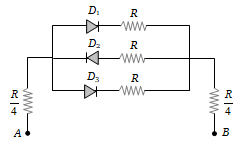(i) – 10 V, – 5V           (ii) – 5V, – 10 V
(iii) – 4V, – 12V

(a) (i) < (ii) < (iii)                       (b) (iii) < (ii) < (i)
(c) (ii) = (iii) < (i)                       (d) (i) = (iii) < (ii)

Concept Questions :-

Applications of PN junction
High Yielding Test Series + Question Bank - NEET 2020

Difficulty Level:

The circuit shown in the following figure contains two diode ${\mathrm{D}}_{1}$ and ${\mathrm{D}}_{2}$ each with a forward resistance of 50 ohms and with infinite backward resistance. If the battery voltage is 6 V, the current through the 100 ohm resistance (in amperes) is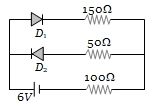(a) Zero                     (b) 0.02
(c) 0.03                     (d) 0.036

Concept Questions :-

PN junction
High Yielding Test Series + Question Bank - NEET 2020

Difficulty Level:

The contribution in the total current flowing through a semiconductor due to electrons and holes are $\frac{3}{4}$ and $\frac{1}{4}$ respectively. If the drift velocity of electrons is $\frac{5}{2}$ times that of holes at this temperature, then the ratio of concentration of electrons and holes is
(a) 6 : 5                 (b) 5 : 6
(c) 3 : 2                 (d) 2 : 3

Concept Questions :-

Types of semiconductors
High Yielding Test Series + Question Bank - NEET 2020

Difficulty Level:

Ge and Si diodes conduct at 0.3 V and 0.7 V respectively. In the following figure if Ge diode connection are reversed, the valve of ${\mathrm{V}}_{0}$ changes by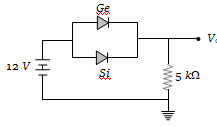(a) 0.2 V                    (b) 0.4 V
(c) 0.6 V                    (d) 0.8 V

Concept Questions :-

PN junction
High Yielding Test Series + Question Bank - NEET 2020

Difficulty Level:

In the circuit shown in the figure, the maximum output voltage ${\mathrm{V}}_{0}$ is :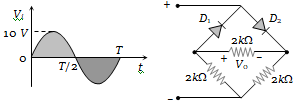1. 0 V                        2. 5 V
3. 10 V                      4. $\frac{5}{\sqrt{2}}$

Concept Questions :-

Rectifier
High Yielding Test Series + Question Bank - NEET 2020

Difficulty Level:

In the following circuit, find ${\mathrm{I}}_{1}$ and ${\mathrm{I}}_{2}$.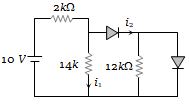(a) 0, 0
(b) 5 mA, 5 mA
(c) 5 mA, 0
(d) 0, 5 mA

Concept Questions :-

Applications of PN junction
High Yielding Test Series + Question Bank - NEET 2020

Difficulty Level:

For the transistor circuit shown below, if $\mathrm{\beta }$ = 100, voltage drop between emitter and base is 0.7 V, then value of ${\mathrm{V}}_{\mathrm{CE}}$ will be :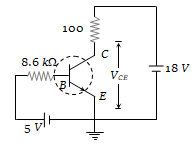(a) 10 V
(b) 5 V
(c) 13 V
(d) 0 V

Concept Questions :-

Transistor
High Yielding Test Series + Question Bank - NEET 2020

Difficulty Level:

The following configuration of gate is equivalent to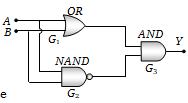(a) NAND
(b) XOR
(c) OR
(d) None of these

Concept Questions :-

Logic gates